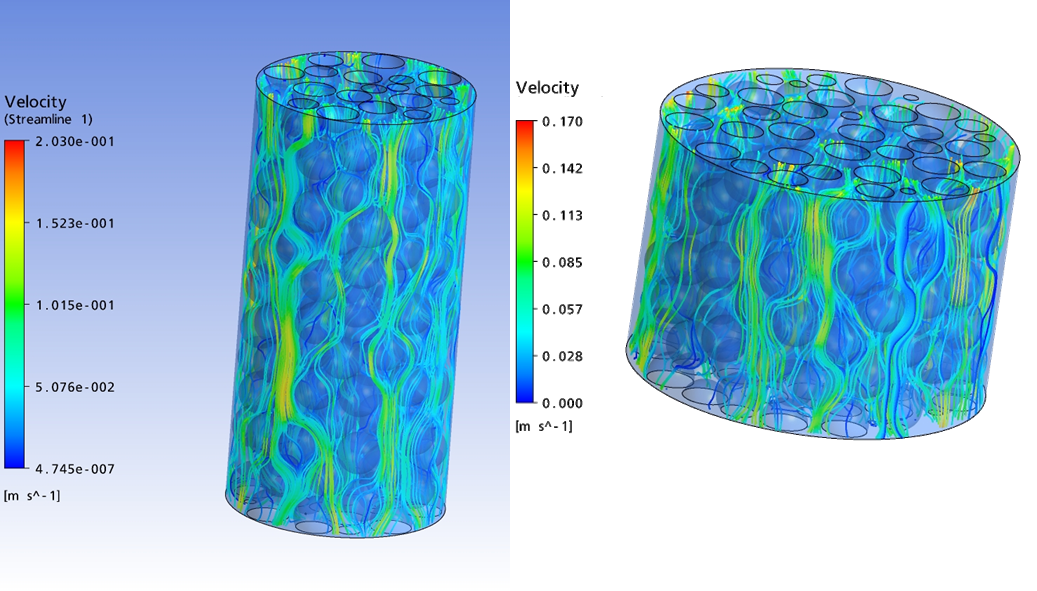# Fluid-dynamic approach (CFD)

CFD methods are based on the solution of differential equations allowing the calculation of velocity, concentration, pressure and temperature fields. These equations are commonly interrelated partial differential equations (PDE), which, in some cases, may be coupled with integro-differential and algebraic equations. Consequently, an analytical solution only exists in few special cases. As a result, for most practical applications, these equations have to be solved numerically. For a numerical solution, approximate algebraic equations are derived, which provide approximate solutions of the PDE for discrete points of the solution region. The determination of these discrete points is called discretisation or grid generation. The derivation of the approximate equations is denoted as discretisation of equations. The system of approximate algebraic equations can be solved on computers. However, the solution of discretised equations is not fully identical with the solution of the original equations. Thus, when interpreting the obtained simulation results, evaluation of discretisation errors should be accomplished.

In our group, CFD methods are used for the simulation of multiphase flows. Such flows appear in a variety of industrial processes; some examples are falling films during condensation and in packed columns or single droplets in the liquid-liquid-extraction. Consequently, fluid dynamics, mass transport and interfacial convection at moving phase interphases of droplets and films represent our main research topics. For the characterization of multiphase flows, the level-set method and the volume of fluid method are mostly used. In both methods, an indicator function identifies the distribution of phases in the computational domain. The aim of our investigations is an improved fundamental understanding of the complex mechanisms at moving phaes boundaries. Furthermore, the obtained results can be used to develop models for the prediction of process performance [1-3].

A further application of CFD is to perform so-called virtual experiments. Here, representative elements of equipment units are investigated numerically, in order to determine process parameters, such as mass or heat transfer coefficients, dispersion coefficients and pressure drop. Thereby, real experiments can be replaced, which are otherwise necessary for the estimation of process parameters. Typical applications of virtual experiments are packed columns , fixed-bed reactors [5-6] and heat exchangers .CFD methods are also applied in mocro-process engineering. In micro-structured apparata, mostly laminar flow is dominating, due to very small unit dimensions. In numerous applications, two continous phases with a defined interface are broucht into contact. This enables a direct and reliable description of two-phase flow in theses apparata.

The possibility to evaluate velocity, pressure, concentration and temperature fields in an apparatus provides a deep insight into the process behaviour, creation of design concepts and geometry optimization [8-10].

  Burghoff, S. and Kenig, E.Y. A CFD model for mass transfer and interfacial phenomena on single droplets. AIChE Journal 52: 4071 - 4078, 2006.  Atmakidis, T. and Kenig, E. Y. A study on the kelvin-helmholtz instability using two different computational fluid dynamics methods. In: The Journal of Computational Multiphase Flows 2(1): 33-45.  Engberg, R.F. and Kenig, E.Y. Numerical simulation of rising droplets in liquid–liquid systems: A comparison of continuous and sharp interfacial force modelsIn: International Journal of Heat and Fluid Flow 50: 16-26, 2014.  Egorov, Y., Menter, F., Kloeker, M. and Kenig, E.Y. On the combination of CFD and rate-based modelling in the simulation of reactive separation processes. Chemical Engineering and Processing 44: 631-644, 2005.  Atmakidis, T. and Kenig, E.Y.CFD-based analysis of the wall effect on the pressure drop in packed beds with moderate tube/particle diameter ratios in the laminar flow regimeIn: Chemical Engineering Journal 155: 404-410, 2009.  Atmakidis, T. and Kenig, E.Y.Numerical analysis of mass transfer in packed-bed reactors with irregular particle arrangementsIn: Chemical Engineering Science 81: 77-83, 2012.  Steube, J., Lautenschleger, A., Piper, M., Böe, D., Weimer, T. and Kenig, E.Y. CFD-based optimisation of spiral wound heat exchanger geometry. In: Chemical Engineering Transactions 29: 1429-1434, 2012.  Chasanis, P., Kenig, E.Y., Hessel, V. and Schmitt, S. Modelling and simulation of a membrane microreactor using computational fluid dynamics. In: Computer-Aided Chemical Engineering, 2008, p. 751-756.  Chasanis, P., Lautenschleger, A. and Kenig, E.Y. Numerical investigation of carbon dioxide absorption in a falling-film micro-contactor. Chemical Engineering Science 65: 1125-1133, 2010  Kenig, E.Y., Ganguli, A., Atmakidis, T. and Chasanis, P.A novel method to capture mass transfer phenomena at free fluid-fluid interfacesIn: Chemical Engineering and Processing 50: 68-76, 2011.